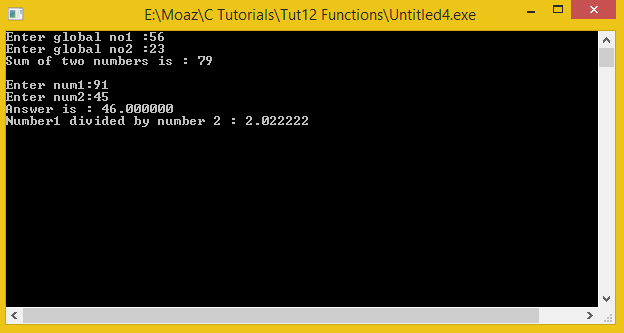# Functions and Global Variables in C

In this part you will learn: 1. Functions 2. Global Variables 3. C syntax 4. Showing output In this tutorial I will teach you how to use functions and global variables in C. What are Functions? Function provides a way in C programming to group instructions as a single unit and give it a name. Function provides many benefits. Using function, a bigger task is divided into many sub-tasks and each sub-task is solved as a function/module. Furthermore, a function can be used/called repeatedly in C program. C programming relies heavily on functions and no meaningful program can be written without the use of functions. For even simple tasks like input and output, we use scanf and printf functions respectively which are declared in the stdio.h header file. e.g void add(int num1,int num2); Here void is the RETURN TYPE , add IS THE FUNCTION NAME, num1 & num2 are the parameters. Every function has these parts but parameters are optional. Three ways of writing functions 1. Functions with no return type. void Functions . Eg: void add(); 2. Functions with parameters Eg : add(int num1,int num2) THERE CAN BE ANY NUMBER OF PARAMETERS 3. Functions with return type Eg : float divide (int num1, int num2) Making functions to make Calculations Basic Step: Open Dev C++ then File > new > source file and start writing the code below.
`#include<stdio.h>#include<conio.h>`
These are the header files that we need for coding. The main header is stdio.h which provides us with most of the functions for coding in C and the second header files is just for using a function which can pause the screen until the user hits any key.
`//Declaring function prototypesvoidinputGlobal(); // for taking input in global variablesvoid add();void subtract(float,float);float divide(float,float); //Declaring global variablesint no1;int no2;`
\ Here in this code we have declared functions prototype before main, this is the basic syntax for calling the functions which are declared outside the main of the program, inside the main these functions are called and defined after the main as shown in the code below. In the second part we have declared variables named ‘no1’ and ‘no2’ as global variables. Global variables are always declared outside the main of the program. Global variables can be used by any function of the class.
`int main(){  float num1,num2;   Float ans=0;   inputGlobal();   add(); //for adding global variables   printf("\n\nEnter num1:");   scanf("%f",&num1);   printf("Enter num2:");   scanf("%f",&num2);   subtract(num1,num2); //subtracting local variables by passing parameters		ans = divide(num1,num2); // dividing local variable by passing parameters and     returning answer	printf("\nNumber1 divided by number 2 : %f ",ans);     getch();     return 0;}`
Here first the local variables are declared and also variable ‘ans’ is initialized as well. Right below the function ‘inputGlobal()’ is called. The syntax for calling a function is main is that we donot have to write return type. Here inputGlobal is the name of the function whereas in the parenthesis the function can take input argument(s) but as the function need no input arguments so the parenthesis are left empty.Whenever we have to give arguments through main , the type of input arguments are not mentioned. Return type is the type of variable like int, float, double etc which the function returns after doing some calculation or work on the variables that have been input in the function. It must be remembered here that in C , a function can return only one variable , suppose if multiple operations are done on the variables which are taken by the function as input but the single variable will be reuturned so for different operations or calculations separate functions have to be defined just like subtract and add function. The subtract function is called in main as ‘subtract(num1,num2);’, here as we see that it has no return type, return type is always mentioned before the name of the function definition(which is done in the later part of the code). Format for calling function in main is as follows functionName(input arguments separated by commas) ; For the subtract and divide function here we have also taken inputs from the users as num1 and num2. These inputs are passed to the function for the relative operation to be performed.
`void inputGlobal(){	printf("Enter global no1 :");	scanf("%d",&no1);	printf("Enter global no2 :");	scanf("%d",&no2);}`
In this piece of code the function inputGlobal which was called in the main is defined, this function basically assign the values to the global variables , as this function need not to return any thing so its return type is void(means empty). As you see that for defining a function its return type is also mentioned. Format for defining a functions is Returntype functionName(input arguments with their variable types separated by commas);
`	void add(){	int answer=0;	answer = no1 + no2;	printf("Sum of two numbers is : %d ",answer);}}`
In the add function simply the two global variable are added to each other , the output is displayed(its not returned), else if the output was to be returned , the return type will be int instead of void.
`void subtract(float n1,float n2){	float ans =0;	ans = n1 - n2;	printf("Answer is : %f ",ans);} float divide(float number1,float number2){	return number1/number2;}`
The subtract function as called in the main takes in two input arguments and subtracts the second number from the first one.The result is simply displayed(printed) on the screen. The divide function also takes in two arguments and perform division , but instead of simply displaying the result , the result is returned to main and is stored in the float type variable ‘ans’ and it is displayed on the screen by simply printing using printf through main. Like other functions the results could be simply displayed on the screen but in case of divide function the result is returned to main and stored in an other variable which is then displayed. Execute > compile then Execute > run Output